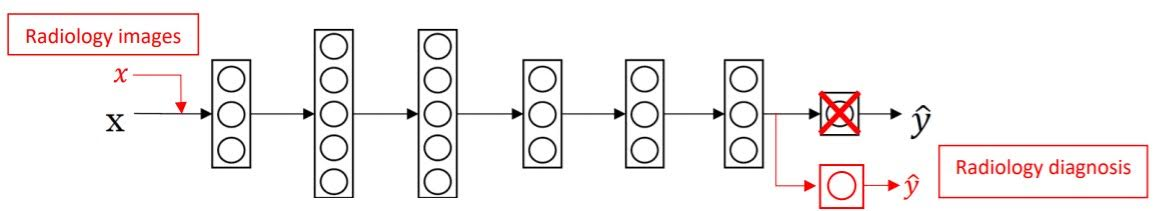# Transfer Learning

Categories:

Updated:

Knowledge gained while solving one problem can be applied to a different but related problem. For example, knowledge gained while learning to recognize cars could apply when trying to recognize trucks. This is called ‘Transfer Learning’.

In neural networks, this ‘knowledge’ is trained weights.

# How do we do transfer learning in neural networks?

This is how you transfer knowledge learned from task A to a new task B.

1. Copy the neural network architecture of task A and apply it to task B.
2. Initialize weights of task B with pre-trained weights of task A.
3. Delete last layer of task B.
4. Add new layers to the tail of neural networks and randomly initialize weights of added layers. You can only add output layer or you can add many layers.
5. With new dataset for task B, train the entire model. If you don’t have enough data, you can make transfered weights untrainable and just train weights of newly added layers.# When should we use transfer learning?

• When task A and B have the same input X
• When there are a lot more data for task A than task B